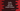# Kotlin program to calculate simple interest with user input values# Calculate simple interest in Kotlin:

In this kotlin programming tutorial, we will learn how to calculate simple interest by using the user provided values. The user will enter the values and the program will calculate the simple interest.

## Algorithm :

Our program will use the below algorithm :

1. Create three variables to store the_ principal amount, rate of interest and number of years_ to calculate the simple interest.
2. Calculate the simple interest and store it in a different variable.
3. Print out the simple interest.
4. Exit.

### Kotlin program :

``````import java.util.Scanner
fun main(args: Array<string>) {
//1
val scanner = Scanner(System.`in`)
//2
print("Enter principal amount : ")
var p:Int = scanner.nextInt()
//3
print("Enter rate of interest : ")
var r:Int = scanner.nextInt()
//4
print("Enter number of years : ")
var n:Int = scanner.nextInt()
//5
var SI:Int = (p*n*r)/100
//6
println("Simple interest : "+SI)
}``````

### Explanation :

The commented numbers in the above program denote the step numbers below :

1. Create one Scanner object to read the user input. This is the same Scanner class object that we use in Java.
2. Ask the user to enter the principal amount. Read it using the scanner object and save it in variable p.
3. Ask the user to enter the rate of interest. Read it and store it in variable_ r_.
4. Ask the user to enter the number of years. Read it and store it in n
5. Calculate the simple interest and store it in the variable SI.
6. Print out the result to the user.

### Sample Output :

``````Enter principal amount : 100
Enter rate of interest : 10
Enter number of years : 10
Simple interest : 100

Enter principal amount : 1200
Enter rate of interest : 12
Enter number of years : 4
Simple interest : 576``````

You can download this program from [here](https://github.com/codevscolor/codevscolor/blob/master/kotlin/simpleinterest.kt)._